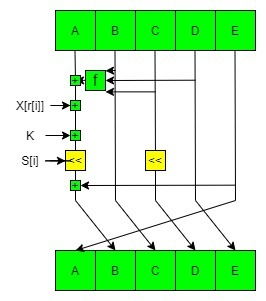# RIPEMD Hash Function

• Difficulty Level : Medium
• Last Updated : 10 Feb, 2020

Hash Function is a function that has a huge role in making a System Secure as it converts normal data given to it as an irregular value of fixed length. We can imagine it to be a Shaker in our homes. When we put data into this function it outputs an irregular value. The Irregular value it outputs is known as “Hash Value”. Hash Values are simply numbers but are often written in Hexadecimal. Computers manage values as Binary. The hash value is also a data and are often managed in Binary.

#### RIPEMD

RIPEMD(RACE Integrity Primitives Evaluation Message Digest) is a group of hash function which is developed by Hans Dobbertin, Antoon Bosselaers and Bart Preneel in 1992. The development idea of RIPEMD is based on MD4 which in itself is a weak hash function. It is developed to work well with 32-bit processors.Types of RIPEMD:

• RIPEMD-128
• RIPEMD-160
• RIPEMD-256
• RIPEMD-320

#### Working

It is a sub-block of the RIPEMD-160 hash algorithm. The message is processed by compression function in blocks of 512 bits and passed through two streams of this sub-block by using 5 different versions in which the value of constant ‘k’ is also different.Different Versions of RIPEMD

• The first RIPEMD was not considered as a good hash function because of some design flaws which leads to some major security problems one of which is the size of output that is 128 bit which is too small and easy to break. In the next version RIPEMD-128, the design flaw is removed but the output is still 128 bit which makes it less secure.
• RIPEMD-160 is the next version which increases the output length to 160 bit and increases the security level of the hash function. This function is designed to work as a replacement for 128-bit hash functions MD4, MD5, and RIPEMD-128.
• RIPEMD-256 and RIPEMD-320 are extension of RIPEMD-128 which provide same security as RIPEMD-160 and RIPEMD-128 which is designed for application which prefer large hash value rather than more security level.

Example 1:

 `# Python program to demonstrate``# RIPEMD `` ` ` ` `import` `hashlib`` ` `# Passing the required algorithm``# as string to the new constructor``x ``=` `hashlib.new(``'ripemd160'``)`` ` `# passing GeeksforGeeks ``# to x() which uses ``# ripemd 160 algorithm for``# hashing``x.update(b``"GeeksForGeeks"``)`` ` `# printing the equivalent hexadecimal``# value. ``print``(``"The hexadecimal equivalent of hash is :"``) ``print``(x.hexdigest())`

Output:

```The hexadecimal equivalent of hash is :
1b4470fb3147534653ddca6d7a1b2109b5449089
```

In the above example, the `new()` constructor takes the algorithm name as a string and creates an object for that algorithm. Then the `update()` method takes a binary string so that it can be accepted by the hash function. The `x()` hash function encodes it and then using `hexdigest()`, hexadecimal equivalent encoded string is printed.

Example 2: Let’s see if we want to find the byte representation of the encoded hash value.

 `# Python program to demonstrate``# RIPEMD `` ` ` ` `import` `hashlib`` ` `# Passing the required algorithm``# as string to the new constructor``x ``=` `hashlib.new(``'ripemd160'``)`` ` `# passing GeeksforGeeks ``# to x() which uses ``# ripemd 160 algorithm for``# hashing``x.update(b``"GeeksForGeeks"``)`` ` `# printing the equivalent hexadecimal``# value. ``print``(``"The byte equivalent of hash is :"``) ``print``(x.digest())`

Output:

```The byte equivalent of hash is :
b'\x1bDp\xfb1GSFS\xdd\xcamz\x1b!\t\xb5D\x90\x89'
```

My Personal Notes arrow_drop_up